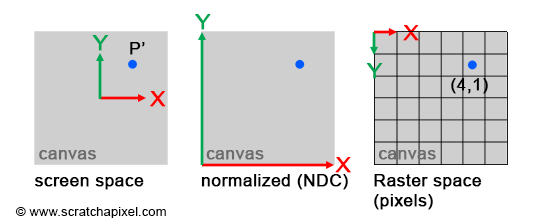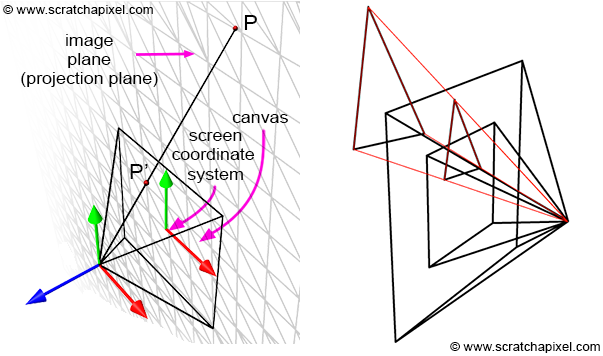$\mathbf{M} = \begin{bmatrix} \color{red}{c_{00}}& \color{red}{c_{01}}&\color{red}{c_{02}}&\color{black}{c_{03}}\\ \color{green}{c_{10}}& \color{green}{c_{11}}&\color{green}{c_{12}}&\color{black}{c_{13}}\\ \color{blue}{c_{20}}& \color{blue}{c_{21}}&\color{blue}{c_{22}}&\color{black}{c_{23}}\\ \color{purple}{c_{30}}& \color{purple}{c_{31}}&\color{purple}{c_{32}}&\color{black}{c_{33}}\\ \end{bmatrix} \begin{array}{l} \rightarrow \quad \color{red} {x-axis}\\ \rightarrow \quad \color{green} {y-axis}\\ \rightarrow \quad \color{blue} {z-axis}\\ \rightarrow \quad \color{purple} {translation}\\ \end{array}$

$\mathbf{R} = \begin{bmatrix} \color{red}{c_{00}}& \color{red}{c_{01}}&\color{red}{c_{02}}\\ \color{green}{c_{10}}& \color{green}{c_{11}}&\color{green}{c_{12}}\\ \color{blue}{c_{20}}& \color{blue}{c_{21}}&\color{blue}{c_{22}}\\ \end{bmatrix} \quad\quad \mathbf{t} = \begin{bmatrix} \color{purple}{c_{30}}& \color{purple}{c_{31}}&\color{purple}{c_{32}} \end{bmatrix}$• 第一行的 $$(\color{red}{c_{00}}, \color{red}{c_{01}}, {\color{red}{c_{02}}})$$ 对应于新坐标系的 x 轴，即其 x 轴上的单位向量在世界坐标系下的表达，
• 第二行的 $$(\color{green}{c_{10}}, \color{green}{c_{11}}, {\color{green}{c_{12}}})$$ 对应于新坐标系的 y 轴，即其 y 轴上的单位向量在世界坐标系下的表达，
• 第三行的 $$(\color{blue}{c_{20}}, \color{blue}{c_{21}}, {\color{blue}{c_{22}}})$$ 对应于新坐标系的 z 轴，即其 z 轴上的单位向量在世界坐标系下的表达，
• 第四行的 $$(\color{purple}{c_{30}}, \color{purple}{c_{31}}, {\color{purple}{c_{32}}})$$ 对应于新坐标系的位置，即其原点（origin）在世界坐标系下的表达。

$\begin{bmatrix} \color{red}{+0.71}&\color{red}{+0.61}&\color{red}{-0.32}&0\\ \color{green}{-0.39}&\color{green}{+0.74}&\color{green}{+0.53}&0\\ \color{blue}{+0.57}&\color{blue}{-0.25}&\color{blue}{+0.77}&0\\ \color{purple}{+0.52}&\color{purple}{+1.25}&\color{purple}{-2.53}&1\\ \end{bmatrix}$\begin{align} &P_{world} = P_{local} * \mathbf{M}\\ &P_{local} = P_{world} * \mathbf{M}^{-1} \end{align}$\frac{BC}{AB} = \frac{B'C'}{AB'}$

$\frac{P.y}{P.z} = \frac{P'.y}{1} \;\;\Rightarrow \;\; P'.y = \frac{P.y}{P.z}$

$P'.x = \frac{P.x}{P.z}$

1. $$P_{camera} = P_{world} * M_{world-to-camera}$$

2. $$P'.x = \dfrac{P_{camera}.x}{P_{camera}.z}, \;\; P'.y = \dfrac{P_{camera}.y}{P_{camera}.z}$$$|P'.x| \leqslant \frac{w}{2}, \;\; |P'.y| \leqslant \frac{h}{2}$

$\begin{array}{l} P'_{normalized}.x = \dfrac{P'.x + w / 2}{ w }\\ P'_{normalised}.y = \dfrac{P'.y + h / 2}{ h } \end{array}$

$\begin{array}{l} P'_{raster}.x = \lfloor{ P'_{normalized}.x * W}\rfloor\\ P'_{raster}.y = \lfloor{ (1 - P'_{normalized}.y) * H}\rfloor \end{array}$

$\text{World Space} \rightarrow \text{Camera Space} \rightarrow \text{Screen Space} \rightarrow \text{NDC Space} \rightarrow \text{Raster Space}.$